# Lateral Face – Definition With Examples

Home » Math-Vocabluary » Lateral Face – Definition With Examples

What do you see when you see a three-dimensional figure?

You don’t just see points and lines. You see its different surfaces, which are called faces.

The bottom surface on which the figure rests is called the base. Some figures have a base at each end, like a prism, which has two bases.

Apart from the base or bases, the remaining faces surrounding the figure are the lateral faces. These lateral surfaces make up the sides of a three-dimensional figure.

## What Is a Lateral Face?

The lateral face of a three-dimensional figure is defined as a face that is on its side instead of on its top or its bottom.

In a cube, the lateral faces are the four square faces that are on its sides, as opposed to the 2 faces that are on the top and bottom of the cube.

A square pyramid has 4 lateral faces and 1 square base.

In a cone, its curved side is continuous and is not separated by edges into faces. So, it does not have any lateral faces. However, the curved side of the cone is considered to be its lateral area.

A cylinder, sphere, and hemisphere do not have lateral faces because their curved sides are not split into different faces.

In a prism, all the faces other than the 2 bases are lateral faces. In any prism, like a triangular prism, hexagonal prism, or decagonal prism, the lateral faces are the surrounding faces on the sides, as opposed to the two bases. These side faces make up the column that connects the two bases of the prism.

For example, in a rectangular prism, the lateral faces are the 4 faces that are on their sides, as opposed to the 2 faces that are on their top and bottom.

## Solved Examples

Example 1: How many lateral faces does a cube have?

Solution: A cube has 6 faces in total: 2 square bases and 4 square side faces.

So, a cube has 4 lateral faces.

Example 2: How many lateral faces does a hexagonal prism have?

Solution: A hexagonal prism has 8 faces in total: 2 hexagonal bases and 6 rectangular faces on its sides.

So, a hexagonal prism has 6 lateral faces.

Example 3: How many lateral faces does a square pyramid have?

Solution: A square pyramid has 5 faces in total: 1 square base and 4 triangular faces on its sides.

So, a square pyramid has 4 lateral faces.

Example 4: Which polygons make up the lateral faces of a triangular prism?

Alt Tag: Hollow triangular prism

Ans: Rectangles are the polygons that make up the sides of a triangular prism.

So, the lateral face of a triangular prism is a rectangle.

## Practice Problems

1

### How many lateral faces does a rectangular prism have?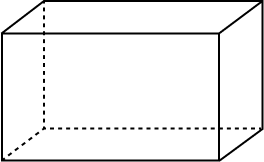6
8
4
3
CorrectIncorrect
A rectangular prism has 6 faces: 2 rectangle bases and 4 rectangle faces on its sides. So, it has 4 lateral faces.
2

### How many lateral faces does a decagonal prism have?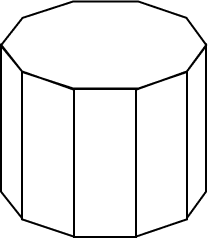12
8
10
6
CorrectIncorrect
A decagonal prism has 12 faces: 2 decagon bases and 8 square faces that make up its sides. So, a decagonal prism has 10 lateral faces.
3

### What are the polygons that make up the lateral faces of a hexagonal prism?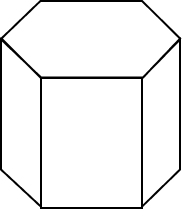Rectangles
Squares
Hexagons
Triangles
CorrectIncorrect
A hexagonal prism has 8 faces: 2 hexagon bases and 6 rectangle faces that make up the sides. So, its lateral faces are rectangles.
4

### What are the lateral faces of a square pyramid?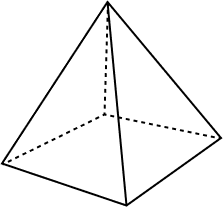Squares
Rectangles
Prisms
Triangles
CorrectIncorrect
A square pyramid has 5 faces: one square base and 4 triangle faces surrounding it on its sides. So, its lateral faces are triangles.
5

### How many faces does a pentagonal prism have without counting its lateral faces?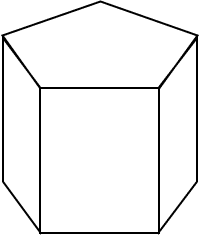5
2
1
3
CorrectIncorrect
A pentagonal prism has 7 faces in total: 2 pentagon bases and 5 rectangular lateral faces. If we don't count the 5 lateral faces, two faces are left.

Lateral faces are the sides of a three-dimensional figure. The faces of a three-dimensional figure include its sides and its base or bases. The lateral faces make up the sides.

In two-dimensional shapes like a square or triangle, the sides are the lines that make up the shape. However, in three-dimensional figures, the sides are the lateral faces.

Not all three-dimensional figures have lateral faces.

Cylinders, hemispheres, spheres, and cones do not have lateral faces because their curved sides are continuous and not split into faces.

However, these curved sides are considered the lateral surface area of these figures.

Lateral surface area refers to the area of the sides of a three-dimensional figure. This includes three-dimensional figures like cylinders and cones that do not have lateral faces but still have lateral surface area.

In the case of a three-dimensional figure that has lateral faces, the surface area of these lateral faces will be the lateral surface area for that figure.